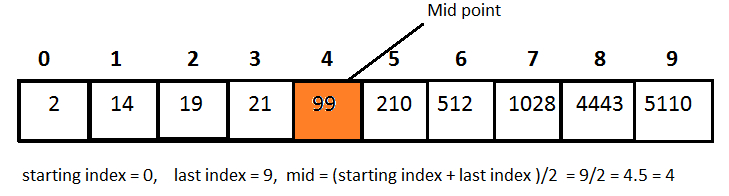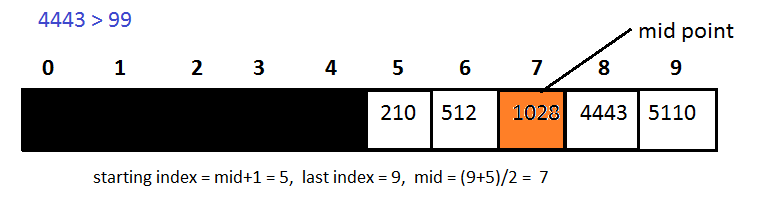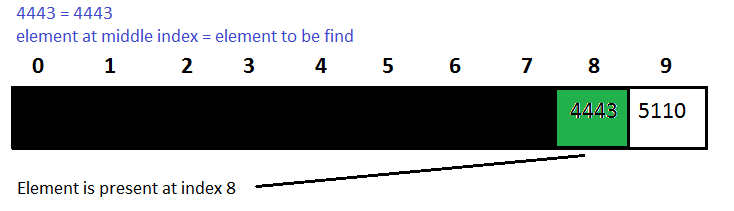# Python Binary Search

Here you will learn about python binary search with program and algorithm.

In linear search, we have to check each node/element. Because of this, time complexity increases. To reduce this time complexity, we use Binary search. In Binary search half of the given array will be ignored after just one comparison.

The main point to be noted is Binary Search only works for sorted array.

If the array is sorted into ascending order, all we have to do is find the middle index of the array and then compare the element at middle index with the element to find. If our given element is greater than the element at middle index then we’ll ignore the left half array and the array will start from the next index of middle index.

On other hand, if the given element is less than the element presents at middle index, then we’ll ignore the right half array and the new array will ends with left one index of middle index.

If the given element is equal to the element presents on the middle index, then search is completed.

In the case, the first index is greater than the last index of array, it means we have been gone through the entire array and the given element is not presented in the array.

## Python Binary Search

### Example:

We’ve a sorted array [2, 14, 19, 21, 99, 210, 512, 1028, 4443, 5110] and the element to be find is 4443.

Step 1:Step 2:Step 3:Step:4### Program for Binary Search in Python

#### Iterative Approach (Using Loop):

Output

element is present at index 8

#### Recursive Approach (Using Recursion):

Output

element is present at index 4

Comment below if you have any queries related to above python binary search algorithm tutorial.

### 6 thoughts on “Python Binary Search”

1.so easy. and understanding for everyone

2.Great post and nice explanation.
One question though…
Why use this syntax for type conversion?
mid = (int)(start_index+last_index)/2
It is used in C language and i’m not sure these are same things.
This works for any function
>>> def f(a):
… return a + 1

>>> (f)(1.5)
2.5
>>>

You’ve could have done this
mid = int((start_index+last_index)/2)
which makes more sense in python, also it seems you are using python2 in which ‘/’ operator returns integer if the operands are integers, so type conversion is unnecessary.

3.how to develop our coding skills

4.loop approach is wrong

5.6.